# 交易策略的色彩优化

4 四月 2019, 08:55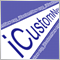0
3 135

### 初始数据

• string aFileName 是包含优化报告的文件名,该文件必须在终端数据目录的 MQL5/Files文件夹中可用。
• SOptimization & aOptimization 是通过引用传递的，函数执行后，从报告中提取的数据将位于该结构中。

SOptimisation 结构:

```struct SOptimization{
string ParameterName[];
SPass Pass[];
};
```

SPass 结构:

```struct SPass{
string Pass;
string Result;
string Profit;
string ExpectedPayoff;
string ProfitFactor;
string RecoveryFactor;
string SharpeRatio;
string Custom;
string EquityDD_perc;
string Parameters[];
};
```

• Pass — 优化过程的编号;
• Result — 优化后的最终余额;
• Profit — 结果利润值;
• ExpectedPayoff — 期望收入值;
• ProfitFactor — 利润因子值;
• RecoveryFactor — 采收系数;
• SharpeRatio — 夏普比率;
• Custom — 一个自定义参数;
• EquityDD_perc — 回撤百分比;
• Parameters[] — 优化的参数数值数组.

• Profitability(盈利能力)，每笔交易的平均利润
• Drawdown(回撤), 相对最大值的净值下降值
• Recovery factor(采收系数)，绝对利润与最大回撤之比

1. 创建 ColorOptimization.mqh 文件，创建彩色报告的所有函数都将位于此文件中。

2. 在 ColorOptimization.mqh 文件的开头关联 HtmlReport.mqh 文件：

`#include <HTMLReport.mqh>`

3. 创建一个新的结构，它继承 SPass 结构的所有栏位，并且在其中加上 factor[] 和 dParameters[] 数组:

```struct SPass2:SPass{
double factor;
double dParameters[];
};
```

4. 为优化数据创建最终结构：

```struct SOptimization2{
string ParameterName[];
SPass2 Pass[];
};
```

5. 创建一个将数据从sOptimeization结构转换为sOptimeization2的函数：

```void ConvertOptimizationStruct(SOptimization & src,SOptimization2 & dst){

ArrayCopy(dst.ParameterName,src.ParameterName);
int cnt=ArraySize(src.Pass);
ArrayResize(dst.Pass,cnt);
for(int i=0;i<cnt;i++){
ArrayCopy(dst.Pass[i].Parameters,src.Pass[i].Parameters);

dst.Pass[i].Pass=src.Pass[i].Pass;
dst.Pass[i].Result=src.Pass[i].Result;
dst.Pass[i].Profit=src.Pass[i].Profit;
dst.Pass[i].ExpectedPayoff=src.Pass[i].ExpectedPayoff;
dst.Pass[i].ProfitFactor=src.Pass[i].ProfitFactor;
dst.Pass[i].RecoveryFactor=src.Pass[i].RecoveryFactor;
dst.Pass[i].SharpeRatio=src.Pass[i].SharpeRatio;
dst.Pass[i].Custom=src.Pass[i].Custom;
dst.Pass[i].EquityDD_perc=src.Pass[i].EquityDD_perc;

dst.Pass[i].factor=StringToDouble(src.Pass[i].Result);
dst.Pass[i].factor=StringToDouble(src.Pass[i].Profit);
dst.Pass[i].factor=StringToDouble(src.Pass[i].ExpectedPayoff);
dst.Pass[i].factor=StringToDouble(src.Pass[i].ProfitFactor);
dst.Pass[i].factor=StringToDouble(src.Pass[i].RecoveryFactor);
dst.Pass[i].factor=StringToDouble(src.Pass[i].SharpeRatio);
dst.Pass[i].factor=StringToDouble(src.Pass[i].Custom);
dst.Pass[i].factor=StringToDouble(src.Pass[i].EquityDD_perc);

int pc=ArraySize(src.Pass[i].Parameters);

ArrayResize(dst.Pass[i].dParameters,pc);

for(int j=0;j<pc;j++){
if(src.Pass[i].Parameters[j]=="true"){
dst.Pass[i].dParameters[j]=1;
}
else if(src.Pass[i].Parameters[j]=="false"){
dst.Pass[i].dParameters[j]=0;
}
else{
dst.Pass[i].dParameters[j]=StringToDouble(src.Pass[i].Parameters[j]);
}
}
}
}
```

```enum EOptimizatrionFactor{
Result=0,
Profit=1,
ExpectedPayoff=2,
ProfitFactor=3,
RecoveryFactor=4,
SharpeRatio=5,
Custom=6,
EquityDD_perc=7,
};
```

6. 创建一个函数，用于将报告文件加载到sOptimeization2结构中，该结构与 HtmlReport.mqh 中的 OptimizerXMLReportToStruct（）类似：

```bool OptimizerXMLReportToStruct2(string aFileName,SOptimization2 & aOptimization){
SOptimization tmp;
if(!OptimizerXMLReportToStruct(aFileName,tmp)){
return(false);
}
ConvertOptimizationStruct(tmp,aOptimization);
return(true);
}
```

### 创建彩色报告

1. 让我们创建一个脚本 ColorOptimization.mq5.

2. 把 ColorOptimization.mqh 与 ColorOptimization.mq5 关联.

`#include <ColorOptimization.mqh>`

3. 在脚本中另外增加外部参数。首先，我们将添加一个指示属性窗口存在的属性，然后我们将添加变量。

`#property script_show_inputs`

```input string               ReportName     =  "*.xml";
input string               OutputName     =  "ColorOptimization1-1.htm";
input EOptimizatrionFactor Factor1        =  Profit;
input EOptimizatrionFactor Factor2        =  EquityDD_perc;
input EOptimizatrionFactor Factor3        =  RecoveryFactor;
input bool                 Factor1Invert  =  false;
input bool                 Factor2Invert  =  true;
input bool                 Factor3Invert  =  false;
input bool                 Sort           =  true;
```

• ReportName — 源优化报告文件的名称;
• OutputName — 由脚本所创建的报告文件的名称;
• Factor1 — 第一个因子，根据该因子确定报告颜色；
• Factor2 — 第二个因子，根据该因子确定报告颜色；
• Factor3 — 第三个因子，根据该因子确定报告颜色；
• Factor1Invert — 反转第一个因子；
• Factor2Invert — 反转第二个因子；
• Factor3Invert — 反转第三个因子；
• Sort — 根据颜色指示对最终报告进行排序；

4. 在脚本的 OnStart（）函数中，我们声明一个sOptimisation2 类型的变量，并接收源报告数据：

```SOptimization2 opt;

if(!OptimizerXMLReportToStruct2(ReportName,opt)){
return;
}
```

5. 由于RGB只是许多不同颜色模型中的一种，因此让我们提供进一步修改库的可能性，特别是添加其他颜色模型。这就是为什么我们将从0到1的抽象值计算开始，而不是计算RGB组件的值。然后我们将这些值转换为从0到255的RGB组件。每个优化过程都使用单独的颜色指示，因此我们需要为SPass2添加三个颜色组件字段。我们不添加三个字段，而是添加一个三元素数组：

`double ColorComponent;`

6. ColorOptimization.mqh 中的 SolveColorComponents() 函数将计算颜色组成部分，应该向函数中传入以下参数:

• SOptimization2 & aOpt — 源优化报告中的数据
• int i1, int i2, int i3 — 源优化报告中数值的索引 (SPass 结构中的 factor 数组)
• bool r1=false, bool r2=false, bool r3=false — 用于反转数值

```void SolveColorComponents(  SOptimization2 & aOpt,
int i1,int i2,int i3,
bool r1=false,bool r2=false,bool r3=false){

double mx={0,0,0};
double mn={DBL_MAX,DBL_MAX,DBL_MAX};

int size=ArraySize(aOpt.Pass);

for(int i=0;i<size;i++){
mx=MathMax(mx,aOpt.Pass[i].factor[i1]);
mx=MathMax(mx,aOpt.Pass[i].factor[i2]);
mx=MathMax(mx,aOpt.Pass[i].factor[i3]);
mn=MathMin(mn,aOpt.Pass[i].factor[i1]);
mn=MathMin(mn,aOpt.Pass[i].factor[i2]);
mn=MathMin(mn,aOpt.Pass[i].factor[i3]);
}

double c1,c2,c3,d;

for(int i=0;i<size;i++){

c1=0;
c2=0;
c3=0;

d=mx-mn;
if(d!=0){
c1=(aOpt.Pass[i].factor[i1]-mn)/d;
}

d=mx-mn;
if(d!=0){
c2=(aOpt.Pass[i].factor[i2]-mn)/d;
}

d=mx-mn;
if(d!=0){
c3=(aOpt.Pass[i].factor[i3]-mn)/d;
}

if(r1)c1=1.0-c1;
if(r2)c2=1.0-c2;
if(r3)c3=1.0-c3;

aOpt.Pass[i].ColorComponent=c1;
aOpt.Pass[i].ColorComponent=c2;
aOpt.Pass[i].ColorComponent=c3;
}

}
```

`SolveColorComponents(opt,Factor1,Factor2,Factor3,Factor1Invert,Factor2Invert,Factor3Invert);`

7. 如果在外部脚本参数中启用排序，则需要计算排序的系数并执行该排序。最佳的优化过程是一个，在此过程中所有参数组合都具有最大值。如果这些参数对应于RGB，则最佳选项是白色。因此，应将排序因子计算为三个分量的算术平均值。

`double SortFactor; `

```void SolveSortFactor(SOptimization2 & aOpt){

int size=ArraySize(aOpt.Pass);

for(int i=0;i<size;i++){
aOpt.Pass[i].SortFactor=0;
for(int j=0;j<3;j++){
aOpt.Pass[i].SortFactor+=aOpt.Pass[i].ColorComponent[j];
}
aOpt.Pass[i].SortFactor/=3;
}
}
```

```void SortFactorSort(SOptimization2 & aOpt){
int size=ArraySize(aOpt.Pass);
for(int i=size-1;i>0;i--){
for(int j=0;j<i;j++){
if(aOpt.Pass[j].SortFactor<aOpt.Pass[j+1].SortFactor){
SPass2 tmp=aOpt.Pass[j];
aOpt.Pass[j]=aOpt.Pass[j+1];
aOpt.Pass[j+1]=tmp;
}
}
}
}
```

```SolveSortFactor(opt);
if(Sort){
SortFactorSort(opt);
}
```

### 带颜色指示的表格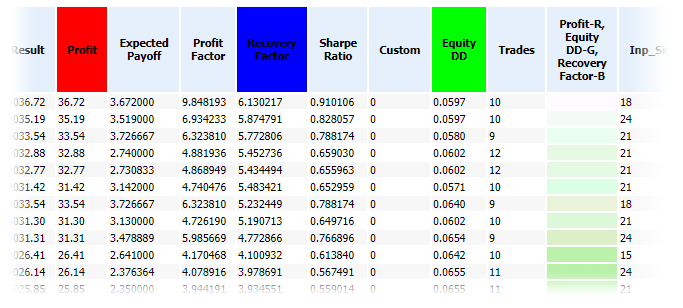TableContent() 函数的完整代码如下:

```string TableContent(SOptimization2 & aOpt,int i1,int i2,int i3){

int size=ArraySize(aOpt.Pass);

int pc=ArraySize(aOpt.ParameterName);

int nc=ArraySize(co_names);

string s="<table>";

s=s+"<tr>";
s=s+"<th>Pass</td>";

for(int i=0;i<nc;i++){
s=s+"<th"+HStyle(i,i1,i2,i3)+">"+co_names[i]+"</th>";
}

for(int j=0;j<pc;j++){
s=s+"<th>"+aOpt.ParameterName[j]+"</th>";
}
s=s+"</tr>";

int r,g,b;

for(int i=0;i<size;i++){

ComponentsToRGB(aOpt.Pass[i].ColorComponent,
aOpt.Pass[i].ColorComponent,
aOpt.Pass[i].ColorComponent,
r,g,b);

s=s+"<tr>";

s=s+"<td>"+aOpt.Pass[i].Pass+"</td>";
s=s+"<td>"+aOpt.Pass[i].Result+"</td>";
s=s+"<td>"+aOpt.Pass[i].Profit+"</td>";
s=s+"<td>"+aOpt.Pass[i].ExpectedPayoff+"</td>";
s=s+"<td>"+aOpt.Pass[i].ProfitFactor+"</td>";
s=s+"<td>"+aOpt.Pass[i].RecoveryFactor+"</td>";
s=s+"<td>"+aOpt.Pass[i].SharpeRatio+"</td>";
s=s+"<td>"+aOpt.Pass[i].Custom+"</td>";
s=s+"<td>"+aOpt.Pass[i].EquityDD_perc+"</td>";

string cs=RGBToStr(r,g,b);
s=s+"<td title='"+cs+"' style='background-color: "+cs+"'>&nbsp</td>";

for(int j=0;j<pc;j++){
s=s+"<td>"+aOpt.Pass[i].Parameters[j]+"</td>";
}

s=s+"</tr>";

}

s=s+"</table>";

return(s);

}
```

```int size=ArraySize(aOpt.Pass);

int pc=ArraySize(aOpt.ParameterName);

int nc=ArraySize(co_names);
```

```string co_names[]={"Result","Profit","Expected Payoff",
"Profit Factor","Recovery Factor",
```

`string s="<table>";`

```s=s+"<tr>";
s=s+"<th>Pass</th>";
```

“Pass”列后面是带参数的列，其中任何一个都可以用来形成颜色指示。确定单元格的HTML代码：

```for(int i=0;i<nc;i++){
s=s+"<th"+HStyle(i,i1,i2,i3)+">"+co_names[i]+"</th>";
}
```

```string HStyle(int i,int i1,int i2,int i3){
if(i==i1)return(" style='background-color: rgb(255,0,0);'");
if(i==i2)return(" style='background-color: rgb(0,255,0);'");
if(i==i3)return(" style='background-color: rgb(0,0,255);'");
return("");
}
```

`s=s+"<th>"+ColorCollHeader(i1,i2,i3)+"</th>";`

```string ColorCollHeader(int i1,int i2,int i3){
return(co_names[i1]+"-R,<br>"+co_names[i2]+"-G,<br>"+co_names[i3]+"-B");
}
```

```for(int j=0;j<pc;j++){
s=s+"<th>"+aOpt.ParameterName[j]+"</th>";
}
s=s+"</tr>";
```

```ComponentsToRGB(aOpt.Pass[i].ColorComponent,
aOpt.Pass[i].ColorComponent,
aOpt.Pass[i].ColorComponent,
r,g,b);
```

ComponentsToRGB() 函数代码:

```void ComponentsToRGB(double c1,double c2,double c3,int & r,int & g,int & b){
r=(int)(c1*255.0);
g=(int)(c2*255.0);
b=(int)(c3*255.0);
}
```

```s=s+"<tr>";

s=s+"<td>"+aOpt.Pass[i].Pass+"</td>";
s=s+"<td>"+aOpt.Pass[i].Result+"</td>";
s=s+"<td>"+aOpt.Pass[i].Profit+"</td>";
s=s+"<td>"+aOpt.Pass[i].ExpectedPayoff+"</td>";
s=s+"<td>"+aOpt.Pass[i].ProfitFactor+"</td>";
s=s+"<td>"+aOpt.Pass[i].RecoveryFactor+"</td>";
s=s+"<td>"+aOpt.Pass[i].SharpeRatio+"</td>";
s=s+"<td>"+aOpt.Pass[i].Custom+"</td>";
s=s+"<td>"+aOpt.Pass[i].EquityDD_perc+"</td>";
```

```string cs=RGBToStr(r,g,b);
s=s+"<td title='"+cs+"' style='background-color: "+cs+"'>&nbsp</td>";
```

RGBToStr() 函数代码:

```string RGBToStr(int r,int g,int b){
return("rgb("+(string)r+","+(string)g+","+(string)b+")");
}
```

```for(int j=0;j<pc;j++){
s=s+"<td>"+aOpt.Pass[i].Parameters[j]+"</td>";
}

s=s+"</tr>";
```

```s=s+"</table>";

return(s);
```

### 优化过参数的平面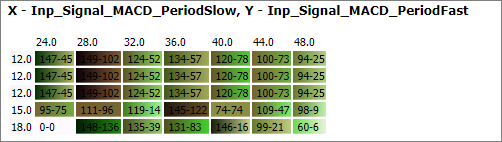```string Color2DPlanes(SOptimization2 & aOpt){
string s="";
int pc=ArraySize(aOpt.ParameterName);
for(int y=0;y<pc;y++){
for(int x=y+1;x<pc;x++){
s=s+Color2DPlane(aOpt,x,y);
}
}
return(s);
}
```

```string Color2DPlane(SOptimization2 & aOpt,int xi,int yi){

double xa[];
double ya[];

int cnt=ArraySize(aOpt.Pass);

ArrayResize(xa,cnt);
ArrayResize(ya,cnt);

for(int i=0;i<cnt;i++){
xa[i]=aOpt.Pass[i].dParameters[xi];
ya[i]=aOpt.Pass[i].dParameters[yi];
}

ArraySort(xa);
ArraySort(ya);

int xc=1;
int yc=1;

for(int i=1;i<cnt;i++){
if(xa[i]!=xa[i-1]){
xa[xc]=xa[i];
xc++;
}
if(ya[i]!=ya[i-1]){
ya[xc]=ya[i];
yc++;
}
}

string s="<hr><h3>X - "+aOpt.ParameterName[xi]+", Y - "+aOpt.ParameterName[yi]+"</h3><table>";

s=s+"<tr>";
s=s+"<td>&nbsp;</td>";
for(int x=0;x<xc;x++){
s=s+"<td>"+(string)xa[x]+"</td>";
}
s=s+"</tr>";
for(int y=0;y<yc;y++){

s=s+"<tr>";

s=s+"<td>"+(string)ya[y]+"</td>";
for(int x=0;x<xc;x++){

double mx=0;
double mn=DBL_MAX;
int mxi=0;
int mni=0;

for(int i=0;i<cnt;i++){
if(aOpt.Pass[i].dParameters[yi]==ya[y] &&
aOpt.Pass[i].dParameters[xi]==xa[x]
){
if(aOpt.Pass[i].SortFactor>mx){
mx=aOpt.Pass[i].SortFactor;
mxi=i;
}
if(aOpt.Pass[i].SortFactor<mn){
mn=aOpt.Pass[i].SortFactor;
mni=i;
}
}
}

int mnr,mng,mnb;
int mxr,mxg,mxb;

ComponentsToRGB(aOpt.Pass[mni].ColorComponent,
aOpt.Pass[mni].ColorComponent,
aOpt.Pass[mni].ColorComponent,
mnr,mng,mnb);

ComponentsToRGB(aOpt.Pass[mxi].ColorComponent,
aOpt.Pass[mxi].ColorComponent,
aOpt.Pass[mxi].ColorComponent,
mxr,mxg,mxb);

string title=RGBToStr(mnr,mng,mnb)+"/"+RGBToStr(mxr,mxg,mxb)+"\n";

int digits[]={2,2,6,6,6,6,6,4,0};

for(int k=0;k<ArraySize(co_names);k++){
title=title+co_names[k]+": "+DoubleToString(aOpt.Pass[mni].factor[k],digits[k])+
"/"+DoubleToString(aOpt.Pass[mxi].factor[k],digits[k])+"\n";
}

s=s+"<td title='"+title+"' style='background: linear-gradient(to right, rgb("+
(string)mnr+","+(string)mng+","+(string)mnb+"), rgb("+
(string)mxr+","+(string)mxg+","+(string)mxb+"));'>&nbsp;"+
(string)mni+"-"+(string)mxi+"</td>";
}
s=s+"</tr>";
}

s=s+"<table>";

return(s);

}
```

```double xa[];
double ya[];

int cnt=ArraySize(aOpt.Pass);

ArrayResize(xa,cnt);
ArrayResize(ya,cnt);

for(int i=0;i<cnt;i++){
xa[i]=aOpt.Pass[i].dParameters[xi];
ya[i]=aOpt.Pass[i].dParameters[yi];
}
```

```ArraySort(xa);
ArraySort(ya);

int xc=1;
int yc=1;

for(int i=1;i<cnt;i++){
if(xa[i]!=xa[i-1]){
xa[xc]=xa[i];
xc++;
}
if(ya[i]!=ya[i-1]){
ya[xc]=ya[i];
yc++;
}
}
```

`string s="<hr><h3>X - "+aOpt.ParameterName[xi]+", Y - "+aOpt.ParameterName[yi]+"</h3><table>";`

```s=s+"<tr>";
s=s+"<td>&nbsp;</td>";
for(int x=0;x<xc;x++){
s=s+"<td>"+(string)xa[x]+"</td>";
}
s=s+"</tr>";
```

`for(int y=0;y<yc;y++){`

```s=s+"<tr>";

s=s+"<td>"+(string)ya[y]+"</td>";
```

`for(int x=0;x<xc;x++){`

```double mx=0;
double mn=DBL_MAX;
int mxi=0;
int mni=0;

for(int i=0;i<cnt;i++){
if(aOpt.Pass[i].dParameters[yi]==ya[y] &&
aOpt.Pass[i].dParameters[xi]==xa[x]
){
if(aOpt.Pass[i].SortFactor>mx){
mx=aOpt.Pass[i].SortFactor;
mxi=i;
}
if(aOpt.Pass[i].SortFactor<mn){
mn=aOpt.Pass[i].SortFactor;
mni=i;
}
}
}
```

```ComponentsToRGB(aOpt.Pass[mni].ColorComponent,
aOpt.Pass[mni].ColorComponent,
aOpt.Pass[mni].ColorComponent,
mnr,mng,mnb);

ComponentsToRGB(aOpt.Pass[mxi].ColorComponent,
aOpt.Pass[mxi].ColorComponent,
aOpt.Pass[mxi].ColorComponent,
mxr,mxg,mxb);
```

```string title=RGBToStr(mnr,mng,mnb)+"/"+RGBToStr(mxr,mxg,mxb)+"\n";

int digits[]={2,2,6,6,6,6,6,4,0};

for(int k=0;k<ArraySize(co_names);k++){
title=title+co_names[k]+": "+DoubleToString(aOpt.Pass[mni].factor[k],digits[k])+
"/"+DoubleToString(aOpt.Pass[mxi].factor[k],digits[k])+"\n";
}
```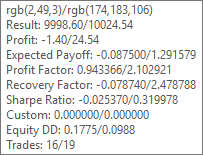```s=s+"<td title='"+title+"' style='background: linear-gradient(to right, rgb("+
(string)mnr+","+(string)mng+","+(string)mnb+"), rgb("+
(string)mxr+","+(string)mxg+","+(string)mxb+"));'>&nbsp;"+
(string)mni+"-"+(string)mxi+"</td>";
```

`s=s+"</tr>";`

```s=s+"<table>";

return(s);
```

```string report=HTMLStart("Color Optimization","style2.css")+
TableContent(opt,Factor1,Factor2,Factor3)+
Color2DPlanes(opt)+HTMLEnd();

```

### 颜色模型的修改

1. 复制 ColorOptimization.mqh 和 ColorOptimization.mq5，并将其保存为ColorOptimization2.mqh 和 ColorOptimization2.mq5。

2. 将两种颜色模型类型的两个常量和一个全局变量添加到ColorOptimization2.mqh中，它将确定颜色模型：

```#define MODEL_RGB 0
#define MODEL_CMY 1

int co_ColorModel;
```

3. 添加枚举和外部变量，用户将使用该变量选择颜色模型：

```enum EColorModel{
RGB=MODEL_RGB,
CMY=MODEL_CMY
};

input EColorModel          ColorModel     =  RGB;
```

`co_ColorModel=ColorModel;`

```void ComponentsToRGB(double c1,double c2,double c3,int & r,int & g,int & b){
if(co_ColorModel==MODEL_RGB){
r=(int)(c1*255.0);
g=(int)(c2*255.0);
b=(int)(c3*255.0);
}
else if(co_ColorModel==MODEL_CMY){
CMYtoRGB(c1,c2,c3,r,g,b);
}
}
```

CMY模型到RGB的转换在单独的函数中实现：

```void CMYtoRGB(double C,double M,double Y,int & R,int & G,int & B){
R=(int)((1.0-C)*255.0);
G=(int)((1.0-M)*255.0);
B=(int)((1.0-Y)*255.0);
}
```

```string HStyle(int i,int i1,int i2,int i3){
if(co_ColorModel==MODEL_RGB){
if(i==i1)return(" style='background-color: rgb(255,0,0);'");
if(i==i2)return(" style='background-color: rgb(0,255,0);'");
if(i==i3)return(" style='background-color: rgb(0,0,255);'");
}
else if(co_ColorModel==MODEL_CMY){
if(i==i1)return(" style='background-color: rgb(0,255,255);'");
if(i==i2)return(" style='background-color: rgb(255,0,255);'");
if(i==i3)return(" style='background-color: rgb(255,255,0);'");
}
return("");
}
```

```string ColorCollHeader(int i1,int i2,int i3){
if(co_ColorModel==MODEL_RGB){
return(co_names[i1]+"-R,<br>"+co_names[i2]+"-G,<br>"+co_names[i3]+"-B");
}
else if(co_ColorModel==MODEL_CMY){
return(co_names[i1]+"-C,<br>"+co_names[i2]+"-M,<br>"+co_names[i3]+"-Y");
}
return "";
}
```

```string cs=RGBToStr(r,g,b);
s=s+"<td title='"+cs+"' style='background-color: "+cs+"'>&nbsp</td>";
```

```string ts="",cs=RGBToStr(r,g,b);

if(co_ColorModel==MODEL_RGB){
ts=cs;
}
else if(co_ColorModel==MODEL_CMY){
ts=CMYToStr(aOpt.Pass[i].ColorComponent,
aOpt.Pass[i].ColorComponent,
aOpt.Pass[i].ColorComponent);
}
s=s+"<td title='"+ts+"' style='background-color: "+cs+"'>&nbsp</td>";
```

`string title=RGBToStr(mnr,mng,mnb)+"/"+RGBToStr(mxr,mxg,mxb)+"\n";`

```string title="";

if(co_ColorModel==MODEL_RGB){
title=RGBToStr(mnr,mng,mnb)+"/"+RGBToStr(mxr,mxg,mxb)+"\n";
}
else if(co_ColorModel==MODEL_CMY){
title=CMYToStr(aOpt.Pass[mni].ColorComponent,
aOpt.Pass[mni].ColorComponent,
aOpt.Pass[mni].ColorComponent)+"/"+
CMYToStr(aOpt.Pass[mxi].ColorComponent,
aOpt.Pass[mxi].ColorComponent,
aOpt.Pass[mxi].ColorComponent)+"\n";
}
```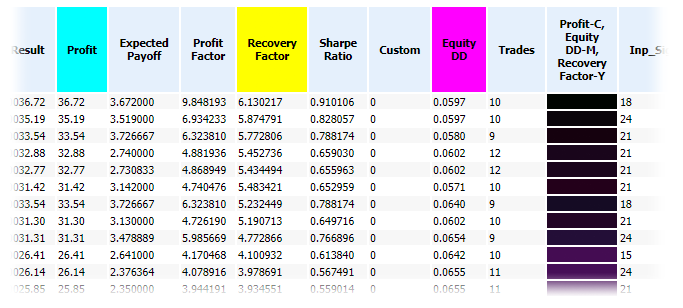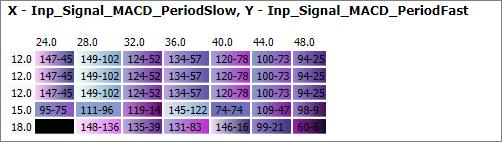### 如何解释颜色指示

RGB中的最佳选项接近白色，而CMY中的最佳选项接近黑色。为了正确解释其他颜色，我们需要了解颜色模型中的各个组件是如何组合的，以及结果颜色是如何形成的。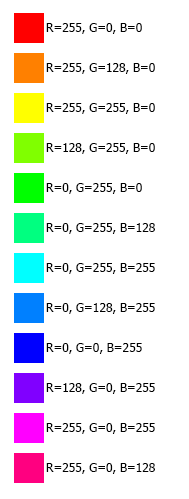RGB模型中的颜色与彩色灯光的添加类似。在CMY模型中，值从白色中减去，因此所有组件的最大值对应于黑色。CMY模型类似于混合颜料：如果没有颜料，我们就有一张白纸；如果混合了太多不同的颜料，你就会得到黑色（或者更确切地说，在处理真正的颜料时是一种肮脏的颜色）。图8. 显示CMY组分的基本组合。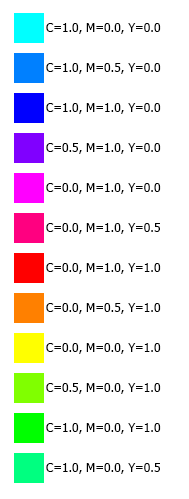### 附件

• HTMLReport.mqh — 报告分析函数的文件 (参见文章 使用 HTML 报告分析交易结果);
• ColorOptimization.mqh — 具有使用RGB模型创建颜色报告的功能的文件；
• ColorOptimization.mq5 — 使用 ColorOptimization.mqh 函数编写脚本，使用示例；
• ColorOptimization2.mqh — 具有创建彩色报告功能的文件，可在RGB和CMY之间进行选择；
• ColorOptimization2.mq5 — 使用 ColorOptimization2.mqh函数编写脚本，使用示例；
• ReportOptimizer-555849.xml — 与文章中使用的优化报告一起归档；
• ColorOptimization.htm — 将通过 ColorOptimization.mq5 脚本获得的报告归档；
• ColorOptimization2.htm — 文件中包含通过 ColorOptimization2.mq5脚本（CMY颜色模型）获得的报告.
• Style2.css — 文件中包含由ColorOptimization.mq5 和 ColorOptimization2.mq5创建的报告的样式。

files.zip (64.67 KB)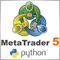MetaTrader 5 与 Python 的集成：接收和发送数据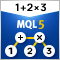利用MQL进行MQL解析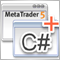基于 .Net 框架和 C# 为 EA 交易和指标开发图形界面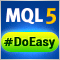轻松快捷开发 MetaTrader 程序的函数库(第一部分)。 概念，数据管理和首期成果